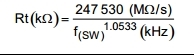This thread has been locked.

If you have a related question, please click the "Ask a related question" button in the top right corner. The newly created question will be automatically linked to this question.

# TPS54618-Q1 RT frequency

Hi

I have a question about equation of RT resistor.

On the data sheet, it is shown at

However it is the following equation actually.(This is the equation of TPS57114)

Which is correct?

Regards,

Koji Hamamoto

• The first equation is correct.  The units of the constant in the numerator is (kohm * kHz^1.027).  For the bottom equation it would be (kohm * kHz^1.0533).  You don't really need to worry about it.  Just use Fsw in kHz to get Rt in kohm.